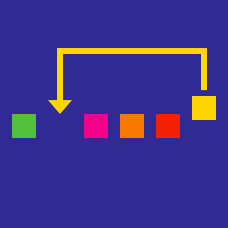Computer Science

# Merge/Quicksort

When two sorted arrays $A$ and $B$ are merged into one big sorted array $C$. What is $C (mod) \ 12$(the remainder of the element with index 400 in $C$ when divided by 12)?

$A = \{ F_i \| 0 \leq i \leq 10000 \}$ $B = \{ i! \| 0 \leq i \leq 1000 \}$

Details and assumptions

• $F_i$ is the $i$th Fibonacci number. $F_0 = 0 \ , F_1 = 1 , F_2 = 1 , ...$

• $n!$ is the factorial of $n$ .$3! = 3\times2\times1$.

The MergeSort(array) method itself is surprisingly simple. The meat of the algorithm is within the auxiliary method Merge. The implementation of mergesort is correct(assuming the Merge method is also correct). It should properly sort any given array of integers. The conditional(Line 5) which handles the base case of the recursion however is noticeably missing. Which of the following code segments correctly fills the empty line?

 1 2 3 4 5 6 7 8 def MergeSort( array ): middle = len(array)/2 #Middle = size(array)/2 A = array[0:middle]# A = array[0..middle B = array[middle::]# B=array[middle..end] #if ... MISSING !!!!! return Merge(A,B) else: return Merge(MergeSort(A),MergeSort(B)) 

Merge-sort is a sorting algorithm that works by splitting a given array $A$ into two sub arrays $\{A_p,\ldots,A_q\}$ and $\{A_{q+1},\ldots,A_r\}$, which are then sorted individually. Once the two arrays are sorted, they are combined to give the overall sorted array.

Merge-sort usually involves two methods: one for merging two sorted arrays and one for the sorting itself.

If we are given a merge function which takes in two sorted arrays and returns the sorted array of their combined elements, merge_sort(A) can be roughly implemented as follows:

 1 2 3 4 5 6 7 merge_sort(A): if len(A) <= 1: return A q = len(A) / 2 A_left = merge_sort(A[0:q+1] A_right = merge_sort(A[q+1:-1]) return merge(A_left, A_right) 

In classic merge-sort we are able to carry out the merging/dividing operation in linear time. For this problem however, assume we are unable to achieve such efficiency and instead the process of merging takes quadratic time($O(n^2)$).

What is the running time of this version of merge-sort?

×